# NCERT Solutions for Class 12 Physics Chapter 2 Electrostatic Potential and Capacitance

In this chapter, we provide NCERT Solutions for Class 12 Physics Chapter 2 Electrostatic Potential and Capacitance for English medium students, Which will very helpful for every student in their exams. Students can download the latest NCERT Solutions for Class 12 Physics Chapter 2 Electrostatic Potential and Capacitance pdf, free NCERT solutions for Class 12 Physics Chapter 2 Electrostatic Potential and Capacitance book pdf download. Now you will get step by step solution to each question. Class 12 physics is a very important subject for entrance exams like IIT JEE, CPMT, etc.

## NCERT Solutions for Class 12 Physics Chapter 2 Electrostatic Potential And Capacitance

Question 1.
Two charges 5 × 10-88 C and -3 × 10-8 C are located 16 cm apart. At what point(s) on the line joining the two charges is the electric potential zero? Take the potential at infinity to be zero. .
Solution:
(i) Let C be the point on the line joining the two charges, where electric potential is zero, thenSo, electric potential is zero at distance of 10 cm from charge of 5 × 10-8 C on line joining the two charges between them. If point C is not between the two charges, then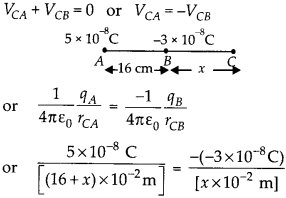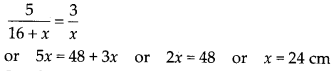So, electric potential is also equal to zero a*a distance of 24 cm from charge of -3 × 10-8 C and at a distance of (24 +16) = 40 cm from charge of 5 × 10-8 C, on the side of charge of -3 × 10-8 C.

Question 2.
A regular hexagon of side 10 cm has a charge 5 μC at each of its vertices. Calculate the potential at the center of the hexagon.

Solution: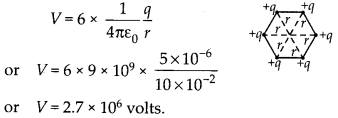Question 3.
Two charges 2 μC and -2 μC are placed at points A and B 6 cm apart.

1. Identify an equipotential surface of the system.
2. What is the direction of the electric field at every point on this surface?

Solution:

1. Since it is an electric dipole, so a plane normal to AB and passing through its mid-point has zero potential everywhere.
2. Normal to the plane in the direction AB.

Question 4.
A spherical conductor of radius 12 cm has a charge of 1.6 × 10-7 C distributed uniformly on its surface. What is the electric field

(a) inside the sphere
(b) just outside the sphere
(c) at a point 18 cm from the centre of the sphere?

Solution: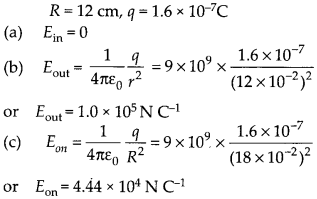Question 5.
A parallel plate capacitor with air between the plates has a capacitance of 8 μF. What will be the capacitance if the distance between the plates is reduced by half, and the space between them is filled with a substance of dielectric constant 6?
Solution:Question 6.
Three capacitors each of capacitance 9 μF are connected in series.
(a) What is the total capacitance of the combination?
(b) What is the potential difference across each capacitors if the combination is connected to a 120 V supply?

Solution:Question 7.
Three capacitors of capacitances 2 μF, 3 μF and 4 μF are connected in parallel.

1. What is the total capacitance of the combination.
2. Determine the charge on each capacitor if the combination is connected to a 100 V supply.

Solution:

1. C = C1 + C2 + C3 = 2 + 3 + 4 or C = 9 μF
2. Since the capacitors are in parallel, so potential difference across each of them is same i.e.

Question 8.
In a parallel plate capacitor with air between the plates, each plate has an area of 6 × 10-3 m2 and the distance between the plates is 3 mm. Calculate the capacitance of the capacitor. If this, capacitor is connected to a 100 V supply, what is the charge on each plate of the capacitor?
Solution: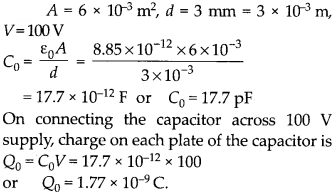Question 9.
Explain what would happen if in the capacitor given in above question, a 3 mm thick mica sheet (of dielectric constant = 6) were inserted between the plates.

(a) While the voltage supply remained connected.
(b) After the supply was disconnected.

Solution:Question 10.
A 12 μF capacitor is connected to a 50 V battery. How much electrostatic energy is stored in the capacitor?
Solution:Question 11.
A 600 μF capacitor is charged by a 200 V supply. It is then disconnected from the supply and is connected to another uncharged 600 μF capacitor. How much electrostatic energy is lost in the process?
Solution:C, = 600 μF, V1 = 200 V, C2 = 600 μF, V2 = 0

Question 12.
Acharge of 8 mC is located at theorigin. Calculate the work done in taking a small charge of – 2 × 10-9 C from a point P(0,0,3 cm) to a point Q (0,4 cm, 0), via a point R (0,6 cm, 9 cm).
Solution:
As electric field is conservative field, so work done in moving a charge in electric field is independent of path chosen to move the charge in electric field and depends only on the electric potential difference between the two end points. So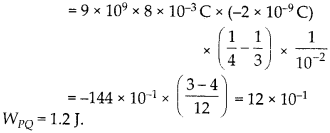Question 13.
A cube of side b has a charge q at each of its vertices. Determine the potential and electric field due to this charge array at the centre of the cube.
Solution:
The length of diagonal of the cube of each side b is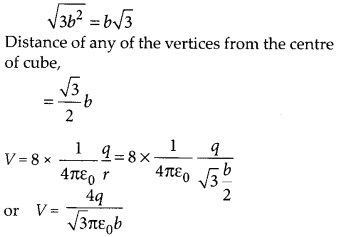E = 0, as electric field at centre due to a charge at any corner of cube is just equal and opposite to that of another charge at diagonally opposite corner of cube.

Question 14.
Two tiny spheres carrying charges 1.5 μC and 2.5 μC are located 30 cm apart. Find the potential and electric field:

(a) at the mid-point of the line joining the two charges, and
(b) at a point 10 cm from this midpoint in a plane normal to the line and passing through the mid-point.

Solution:
(a) At mid point C of line joining two charges, electric potential is Vc = VCA + VCB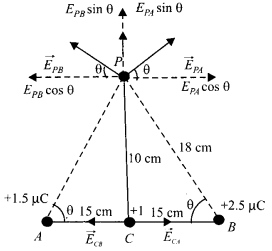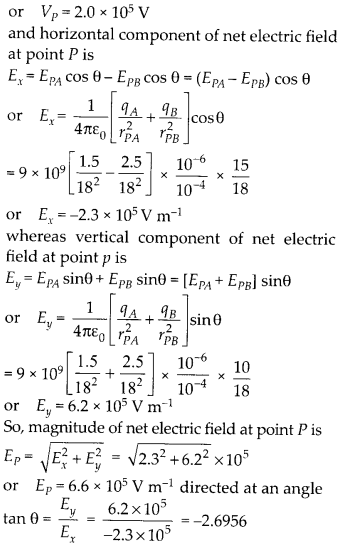Question 15.
A spherical conducting shell of inner radius r, and outer radius r2 has a charge Q.

1. A charge q is placed at the centre of the shell. What is the surface charge density on the inner and outer surfaces of the shell?
2. Is the electric field inside a cavity (with no charge) zero, even if the shell is not spherical, but has any irregular shape? Explain.

Solution:

1. Surface charge density on the inner surface of shell is2. The electric flux linked with any closed surface S inside the conductor is zero as electric field inside conductor is zero.
So, by Gauss’s theorem net charge enclosed by closed surface S is also zero i.e.,$q_{ net }=0$
So, if there is no charge inside the cavity, then there cannot be any charge on the inner surface of the shell and hence electric field inside the cavity will be zero, even though the shell may not be spherical.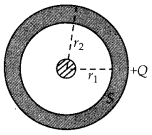Question 16.
Show that the normal component of electrostatic field has a discontinuity from one side of a charged surface to another given by$\left( \overrightarrow { E } _{ 2 }-\overrightarrow { E } _{ 1 } \right) .\hat { n } =\frac { \sigma }{ \varepsilon _{ 0 } }$ where n is a unit vector normal eo to the surface at a point and o is the surface charge density at that point. (The direction of n is from side 1 to side 2). Hence show that just
outside a conductor, the electric field is$\sigma \frac { \hat { n } }{ \varepsilon _{ 0 } }$.
(b) Show that the tangential component of electrostatic field is continuous from one side of a charged surface to another.
Solution:
Normal component of eDctric field intensity due to a thin infinite plane sheet of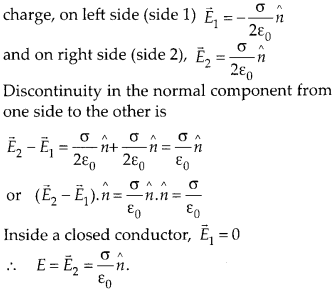(b) To show that the tangential component of electrostatic field is continuous from one side of a charged surface to another, we use the fact that work done by electrostatic field on a closed loop is zero.

Question 17.
A long charged cylinder of linear charge density k is surrounded by a hollow co-axial conducting cylinder. What is the electric field in the space between the two cylinders?
Solution:
In Figure, A is a long charged cylinder of linear charge density k, length l and radius a. A hollow co-axial conducting cylinder B of of length l and radius b surrounds A. The charge q = kl spreads uniformly on the outer surface of A. It induces – q charge on the cylinder B, which spreads on the inner surface of B. An electric field E is produced in the space between the two cylinders, which is directed radially outwards. Let us consider a co-axial cylindrical Gaussian surface of radius r. The electric flux through the cylindrical Gaussian surface is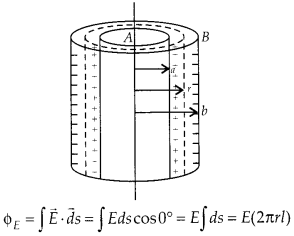The electric flux through the end faces of the cylindrical Gaussian surface is zero, as E is parallel to them. According to Gauss’sQuestion 18.
In a hydrogen atom, the electron and proton are bound at a distance of about 0.53 A:
(a) Estimate the potential energy of the system in eV, taking the zero of the potential energy at infinite separation of the electron from proton.
(b) What is the minimum work required to free the electron, given that its kinetic energy in the orbit is half the magnitude of potential energy obtained in (a)?
(c) What are the answers to (a) and (b) above if the zero of potential energy is taken at 1.06 A separation?
Solution: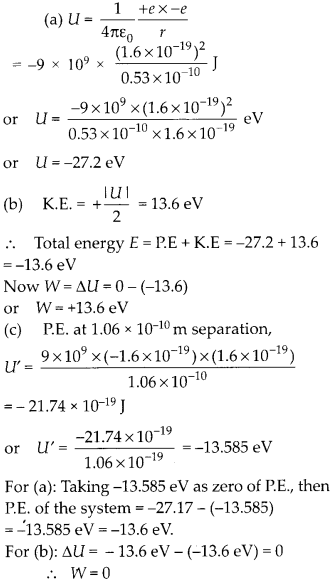Question 19.
If one of the two electrons of a H2 molecule is removed, we get a hydrogen molecular ion${ H }_{ 2 }^{ + }$. In the ground state of an${ H }_{ 2 }^{ + }$, the two protons are separated by roughly 1.5 A, and the electron is roughly 1 A from each proton. Determine the potential energy of the system. Specify your choice of the zero of potential energy.
Solution:
It is a system of three point charges and the potential energy stored is this system of charges iswith zero potential energy at infinity.

Question 20.
TWO charged conducting spheres of radii a and b are connected to each other by a wire. What is the ratio of electric fields at the surfaces of the two spheres? Use the result obtained to explain why charge density on the sharp and pointed ends of a conductor is higher than on its flatter portions.
Solution:
As the two spheres are connected to each other by wire, so they have same electric potential i.e.,Va = Vb

if b > a, then Ea > Eb
i.e. sphere with smaller radius produces more electric field on its surface. Hence, the charge density on the sharp and pointed ends of conductor is higher than on its flatter portions.

Question 21.
Two charges -q and +q are located at points (0,0, -a) and (0,0, a), respectively.

(a) What is the electrostatic potential at the points (0,0, z) and (x, y, 0)?
(b) Obtain the dependence of potential on the distance r of a point from the origin when r/a »1.
(c) How much work is done in moving a small test charge from the point (5, 0, 0) to (-7, 0, 0) along the x-axis? Does the

answer change if the path of the test charge between the some points is not along the x-axis?
Solution:
(a) The two point charges form an electric dipole of moment p = q 2a directed along + z-axis. Point A (0, 0, z) lies on the axis of electric dipole, so electric potential at point A isPoint B (x, y, 0) lies on the equatorial plane of electric dipole, so electric potential at point B is zero i.e. VB = 0
(b) Electric potential at any point on the axis of electric dipole at distance r from its centre is(c) As both the points C (5, 0, 0) and D (-7, 0, 0) lie on the perpendicular bisector of electric dipole, so electric potential at both the points is zero. Hence work done in moving the charge from C to D is
WCD = q0 [VD – VC] × O or WCD = O
This work done will remain equal to zero even if the path of the test charge between the same points is changed, as electric field is conservative field and work done in moving a charge between the two points in electric field is independent of the path chosen to move the charge.

Question 22.
Figure shows a charge array known as an electric quadrupole. For a point on the axis of quadrupole, obtain the dependence of potential on r for r/a >> 1, and contrast your results with that due to an electric dipole, and an electric monopole (i.e., a single charge).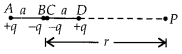Solution:Question 23.
An electrical technician requires a capacitance of 2 μF in a circuit across a potential difference of 1 kV. A large number of 1 μF capacitors are available to him each of which can withstand a potential difference of not more than 400 V. Suggest a possible arrangement that requires the minimum number of capacitors.
Solution:
Minimum number of capacitors that must be connected in series in a row are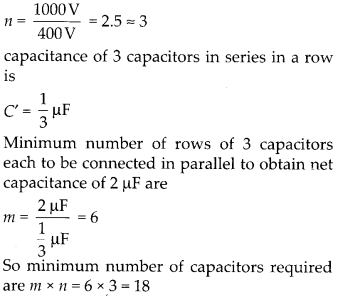Question 24.
What is the area of the plates of a 2 F parallel plate capacitor, given that the separation between the plates is 0.5 cm?

Solution: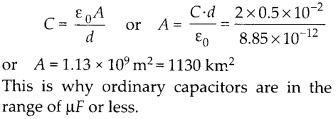Question 25.
Obtain the equivalent capacitance of the network in figure. For a 300 V supply, determine the charge and voltage across each capacitor.Solution:Question 26.
The plates of a parallel plate capacitor have an area of 90 cm2 eacn and are separated by 2.5 mm.The capacitor is charged by connecting it to a 400 V supply
(a) How much electrostatic energy is stored by the capacitor?
(b) View this energy as stored in the electro-static field between the plates, and obtain the energy per unit volume u. Hence arrive at a relation between u and the magnitude of electric field E between the plates.

Solution: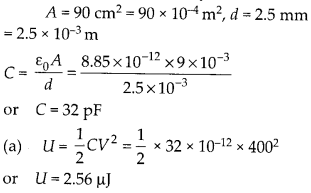Question 27.
A 4 μF capacitor is charged by a 200 V supply. It is then disconnected from the supply, and is connected to another uncharged 2 μF capacitor. How much electrostatic energy of the first capacitor is lost in the form of heat and electromagnetic radiation?

Solution: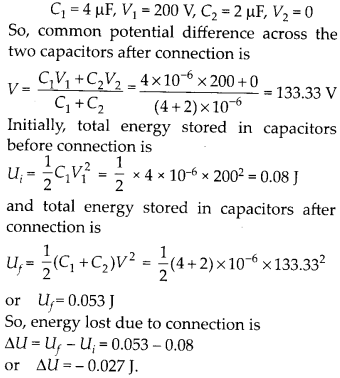Question 28.
Show that the force on each plate of a parallel plate capacitor has magnitude equal to$\frac { 1 }{ 2 }$ QE, where Q is the charge on the capacitor, and E is the magnitude of electric field between the plates. Explain the origin of the factor$\frac { 1 }{ 2 }$.
Solution:
Magnitude of electric field between the
plates of charged capacitor is$E\frac { \sigma }{ \varepsilon _{ 0 } }$ However, magnitude of electric field of one plate or! the other plate of charged capacitor is$E_{ 1 }\frac { \sigma }{ 2\varepsilon _{ 0 } }$ So, force on the one plate of charged capacitor due to the other is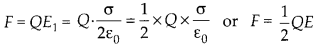The factor$\frac { 1 }{ 2 }$ is because the electric field of one 2 plate on the other plate of charged capacitor is$\frac { 1 }{ 2 }$ of the resultant electric field E between the 2 plates of charged capacitor.

Question 29.
A spherical capacitor consists of two concentric spherical conductors held in position by suitable insulating supports. Show that the capacitance of a spherical capacitor is given by$C=\frac { 4\Pi \varepsilon _{ 0 }r_{ 1 }r_{ 2 } }{ r_{ 1 }-r_{ 2 } }$ Where r1 and r2 are the radii of outer and inner spheres, respectively.
Solution:
Let + Q be the charge on outer spherical shell A of radius r, and + Q be the charge on inner spherical shell B of radius r2. Then electric potential on shell A isThis gives the capacitance of the spherical capacitor.

Question 30.
A spherical capacitor has an inner sphere of radius 12 cm and outer sphere of radius 13 cm. The outer sphere is earthed and the inner sphere is given a charge of 2.5 μC. The space between the concentric spheres is filled with liquid of dielectric constant 32.

(a) Determine the capacitance of the capacitor.
(b) What is the potential of the inner sphere.
(c) Compare the capacitance of this capacitor with that of an isolated sphere of radius 12 cm. Explain why the latter is much smaller.
Solution: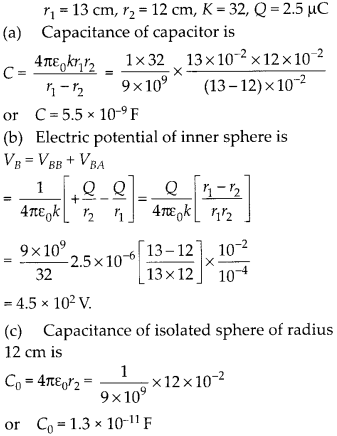Here C > C0, because a single conductor A can be charged to a electric potential till it reaches the breakdown value of surroundings. But when another earthed metallic conductor B is ; brought near it, negative charge induced on it decreases the electric potential on A, hence more charge can not be stored on A.

Question 31.

1. Two large conducting spheres carrying charges Q1 and Q2 are brought close to each other, is the magnitude of electrostatic force between them exactly given by$\frac { Q_{ 1 }Q_{ 2 } }{ 4\pi \varepsilon _{ 0 }r^{ 2 } }$ r is the distance between their centres?
2. If coulomb’s law involved 1 /r3 dependence (instead of 1/r2), would Gauss’s law be still true?
3. A small test charge is released at rest at a point in an electrostatic field configuration. Will it travel along the field line passing through that point?
4. What is the work done by the field of a nucleus in a complete circular orbit of the electron? What if the orbit is elliptical?
5. We know that electric field is discontinuous across the surface of a charged conductor. Is electric potential also discontinuous there?
6. What meaning would you give to the capacitance of a single conductor?
7. Guess a possible reason why water has a much greater dielectric constant {- 80) then say, mica (= 6).

Solution:

1. No, because coulomb’s law holds good only for point charges. ‘
2. No, because in that case electric flux linked with the closed surface will also become dependent on V other than charge enclosed by it.
3. No, it will travel along the field line only if it is a straight line.
4. Zero, whatever may be the shape of orbit may be. It is because work done in moving a charge in closed path in electric field is zero, as electric field is a conservative field.
5. No, electric potential is continuous
there. As E = 0, so$\frac { dV }{ dr }$ = 0 or V = constant.
6. It means that a single conductor is a capacitor whose other plate can be considered to be at infinity.
7. A water molecule is a polar molecule with non-zero electric dipole moment, however mica does not have polar molecules. So, dielectric constant of water is high.

Question 32.
A cylindrical capacitor has two co-axial cylinders of length 15 cm and radii 1.5 cm and 1.4 cm. The outer cylinder is earthed and the inner cylinder is given a charge of 3.5 μC. Determine the capacitance of the system and the potential of the inner cylinder. Neglect end effects (i.e. bending of the field lines at the ends).

Solution: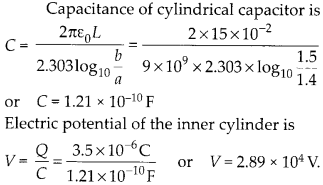Question 33.
A parallel plate capacitor is to be designed with a voltage rating 1 kV, using a material of dielectric constant 3 and dielectric strength about 10V m-1. For safety, we should like the field never to exceed, say 10% of the dielectric strength. What minimum area of the plates is required to have a capacitance of 50 μF?

Solution:Question 34.
Describe schematically the equipotential surfaces corresponding to

• A constant electric field in the z-direction.
• a field that uniformly increases in magnitude but remains in a constant (say, z) direction.
• a single positive charge at the origin, and
• a uniform grid consisting of long equally spaced parallel charged wires in a plane.

Solution:

• Planes parallel to x-y plane or normal to the electric field in z-direction.
• Planes parallel to x-y plane or normal to the electric field in z-direction, but the planes having different fixed potentials will become closer with increase in electric field intensity.
• Concentric spherical surfaces with their centres at origin.
• A time dependent changing shape nearer to grid, and at far off distances from the grid, it slowly becomes planar and parallel to the grid.

Question 35.
In a Van-de-Graff type generator, a spherical metal shell is to be a 15 × 106 V electrode. The dielectric strength of the gas surrounding the electrode is 5 × 10V m1. What is the minimum radius of the spherical shell required?
Solution: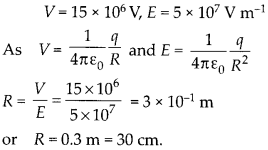Question 36.
A small sphere of radius r1 and charge q1 is enclosed by a spherical shell of radius r2 and charge q2. Show that if q: is positive, charge will necessary flow from the sphere to the shell (when the two are connected by a wire) no matter what the charge q2 on the shell is.
Solution:
The potential on inner small sphere is VA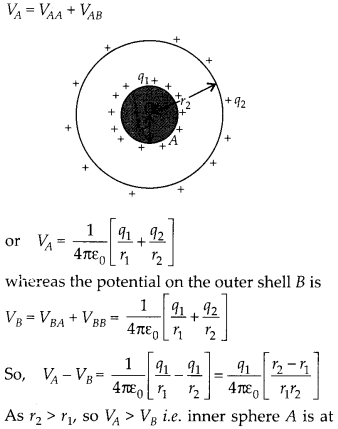higher potential than outer conducting shell B, for any value of charge qv So, when inner sphere A is connected to outer shell B, then charge will flow from inner sphere A to outer shell B, until electric potentials on them is same i.e.
VA – VB = 0 or q1 = 0 [As r1 # r2]

So, charge ql given to sphere A will flow on the shell B, no matter what the charge on the shell B is.

Question 37.

1. The top of the atmosphere is at about 400 kV with respect to the surface of the earth, corresponding to an electric field that decreases with altitude. Near the surface of the earth, the field is about 100 V m-1. Why then do we not get an electric shock as we step out of our house into the open? (Assume the house to be a steel cage, so there is no field inside).
2. A man fixes outside house one evening a two metre high insulating slab carrying on its top a large aluminium sheet of area 1 m2. Will he get an electric shock if he touches the metal sheet next morning?
3. The discharging current in the atmosphere due to the small conductivity of air is known to be 1800 A on an average over the globe. Why then does the atmosphere not discharge itself completely in due course and become electrically neutral? In other words, what keeps the atmosphere charged?
4. What are the forms of energy into which the electrical energy of the atmosphere is dissipated during a lightning?

Solution:

1. Our body and the ground are at same electric potential. As we step out into the open, the original equipotential surfaces of open air change, keeping our head and the ground at the same potential.
2. Yes, it is because the aluminium sheet gets charged due to discharging current and raises to the extent depending on the capacitor formed by the sheet and the ground.
3. The atmosphere of earth gets continuously charged due to lightning, thunderstorms but simultaneously it gets discharged through normal weather zones. This keeps the system balanced.
4. Light, sound and heat energy. Light energy in lightning and heat and sound energy in the accompanying thunder.

All Chapter NCERT Solutions For Class12 Physics

—————————————————————————–

All Subject NCERT Solutions For Class12

*************************************************

Remark:

I think you got complete solutions for this chapter. If You have any queries regarding this chapter, please comment on the below section our subject teacher will answer you. We tried our best to give complete solutions so you got good marks in your exam.

If these solutions have helped you, you can also share Careerkundali.in to your friends.

Best of Luck!!